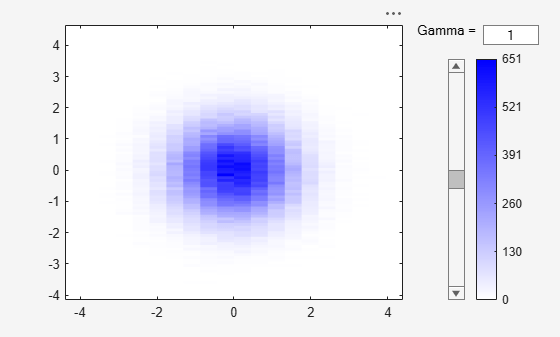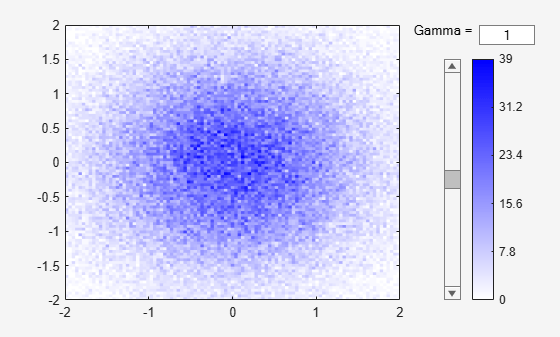# binScatterPlot

Scatter plot of bins for tall arrays

## Syntax

``binScatterPlot(X,Y)``
``binScatterPlot(X,Y,nbins)``
``binScatterPlot(X,Y,Xedges,Yedges)``
``binScatterPlot(X,Y,Name,Value)``
``h = binScatterPlot(___)``

## Description

example

````binScatterPlot(X,Y)` creates a binned scatter plot of the data in `X` and `Y`. The `binScatterPlot` function uses an automatic binning algorithm that returns bins with a uniform area, chosen to cover the range of elements in `X` and `Y` and reveal the underlying shape of the distribution.```

example

````binScatterPlot(X,Y,nbins)` specifies the number of bins to use in each dimension.```

example

````binScatterPlot(X,Y,Xedges,Yedges)` specifies the edges of the bins in each dimension using the vectors `Xedges` and `Yedges`.```

example

````binScatterPlot(X,Y,Name,Value)` specifies additional options with one or more name-value pair arguments using any of the previous syntaxes. For example, you can specify `'Color'` and a valid color option to change the color theme of the plot, or `'Gamma'` with a positive scalar to adjust the level of detail.```
````h = binScatterPlot(___)` returns a `Histogram2` object. Use this object to inspect properties of the plot.```

## Examples

collapse all

Create two tall vectors of random data. Create a binned scatter plot for the data.

When you perform calculations on tall arrays, MATLAB® uses either a parallel pool (default if you have Parallel Computing Toolbox™) or the local MATLAB session. To run the example using the local MATLAB session when you have Parallel Computing Toolbox, change the global execution environment by using the `mapreducer` function.

```mapreducer(0) X = tall(randn(1e5,1)); Y = tall(randn(1e5,1)); binScatterPlot(X,Y)```
```Evaluating tall expression using the Local MATLAB Session: - Pass 1 of 2: Completed in 0.93 sec - Pass 2 of 2: Completed in 0.25 sec Evaluation completed in 2.5 sec Evaluating tall expression using the Local MATLAB Session: - Pass 1 of 1: Completed in 0.19 sec Evaluation completed in 0.27 sec ```The resulting figure contains a slider to adjust the level of detail in the image.

Specify a scalar value as the third input argument to use the same number of bins in each dimension, or a two-element vector to use a different number of bins in each dimension.

When you perform calculations on tall arrays, MATLAB® uses either a parallel pool (default if you have Parallel Computing Toolbox™) or the local MATLAB session. To run the example using the local MATLAB session when you have Parallel Computing Toolbox, change the global execution environment by using the `mapreducer` function.

`mapreducer(0)`

Plot a binned scatter plot of random data sorted into 100 bins in each dimension.

```X = tall(randn(1e5,1)); Y = tall(randn(1e5,1)); binScatterPlot(X,Y,100)```
```Evaluating tall expression using the Local MATLAB Session: - Pass 1 of 1: Completed in 0.49 sec Evaluation completed in 0.68 sec Evaluating tall expression using the Local MATLAB Session: - Pass 1 of 1: Completed in 0.14 sec Evaluation completed in 0.22 sec ```Use 20 bins in the x-dimension and continue to use 100 bins in the y-dimension.

`binScatterPlot(X,Y,[20 100])`
```Evaluating tall expression using the Local MATLAB Session: - Pass 1 of 1: Completed in 0.08 sec Evaluation completed in 0.14 sec Evaluating tall expression using the Local MATLAB Session: - Pass 1 of 1: Completed in 0.069 sec Evaluation completed in 0.091 sec ```Plot a binned scatter plot of random data with specific bin edges. Use bin edges of `Inf` and `-Inf` to capture outliers.

When you perform calculations on tall arrays, MATLAB® uses either a parallel pool (default if you have Parallel Computing Toolbox™) or the local MATLAB session. To run the example using the local MATLAB session when you have Parallel Computing Toolbox, change the global execution environment by using the `mapreducer` function.

`mapreducer(0)`

Create a binned scatter plot with 100 bin edges between `[-2 2]` in each dimension. The data outside the specified bin edges is not included in the plot.

```X = tall(randn(1e5,1)); Y = tall(randn(1e5,1)); Xedges = linspace(-2,2); Yedges = linspace(-2,2); binScatterPlot(X,Y,Xedges,Yedges)```
```Evaluating tall expression using the Local MATLAB Session: - Pass 1 of 1: Completed in 0.86 sec Evaluation completed in 1.2 sec ```Use coarse bins extending to infinity on the edges of the plot to capture outliers.

```Xedges = [-Inf linspace(-2,2) Inf]; Yedges = [-Inf linspace(-2,2) Inf]; binScatterPlot(X,Y,Xedges,Yedges)```
```Evaluating tall expression using the Local MATLAB Session: - Pass 1 of 1: Completed in 0.24 sec Evaluation completed in 0.36 sec ```Plot a binned scatter plot of random data, specifying `'Color'` as `'c'`.

When you perform calculations on tall arrays, MATLAB® uses either a parallel pool (default if you have Parallel Computing Toolbox™) or the local MATLAB session. To run the example using the local MATLAB session when you have Parallel Computing Toolbox, change the global execution environment by using the `mapreducer` function.

```mapreducer(0) X = tall(randn(1e5,1)); Y = tall(randn(1e5,1)); binScatterPlot(X,Y,'Color','c')```
```Evaluating tall expression using the Local MATLAB Session: - Pass 1 of 2: Completed in 0.48 sec - Pass 2 of 2: Completed in 0.16 sec Evaluation completed in 1.4 sec Evaluating tall expression using the Local MATLAB Session: - Pass 1 of 1: Completed in 0.11 sec Evaluation completed in 0.15 sec ```## Input Arguments

collapse all

Data to distribute among bins, specified as separate arguments of tall vectors, matrices, or multidimensional arrays. `X` and `Y` must be the same size. If `X` and `Y` are not vectors, then `binScatterPlot` treats them as single column vectors, `X(:)` and `Y(:)`.

Corresponding elements in `X` and `Y` specify the x and y coordinates of 2-D data points, `[X(k),Y(k)]`. The underlying data types of `X` and `Y` can be different, but `binScatterPlot` concatenates these inputs into a single `N`-by-`2` tall matrix of the dominant underlying data type.

`binScatterPlot` ignores all `NaN` values. Similarly, `binScatterPlot` ignores `Inf` and `-Inf` values, unless the bin edges explicitly specify `Inf` or `-Inf` as a bin edge.

Note

If `X` or `Y` contain integers of type `int64` or `uint64` that are larger than `flintmax`, then it is recommended that you explicitly specify the bin edges.`binScatterPlot` automatically bins the input data using double precision, which lacks integer precision for numbers greater than `flintmax`.

Data Types: `single` | `double` | `int8` | `int16` | `int32` | `int64` | `uint8` | `uint16` | `uint32` | `uint64` | `logical`

Number of bins in each dimension, specified as a positive scalar integer or two-element vector of positive integers. If you do not specify `nbins`, then `binScatterPlot` automatically calculates how many bins to use based on the values in `X` and `Y`.

• If `nbins` is a scalar, then `binScatterPlot` uses that many bins in each dimension.

• If `nbins` is a vector, then `nbins(1)` specifies the number of bins in the x-dimension and `nbins(2)` specifies the number of bins in the y-dimension.

Example: `binScatterPlot(X,Y,20)` uses 20 bins in each dimension.

Example: `binScatterPlot(X,Y,[10 20])` uses 10 bins in the `x`-dimension and 20 bins in the `y`-dimension.

Bin edges in x-dimension, specified as a vector. `Xedges(1)` is the first edge of the first bin in the x-dimension, and `Xedges(end)` is the outer edge of the last bin.

The value `[X(k),Y(k)]` is in the `(i,j)`th bin if `Xedges(i)``X(k)` < `Xedges(i+1)` and `Yedges(j)``Y(k)` < `Yedges(j+1)`. The last bins in each dimension also include the last (outer) edge. For example, `[X(k),Y(k)]` falls into the `i`th bin in the last row if `Xedges(end-1)``X(k)``Xedges(end)` and `Yedges(i)``Y(k)` < `Yedges(i+1)`.

Data Types: `single` | `double` | `int8` | `int16` | `int32` | `int64` | `uint8` | `uint16` | `uint32` | `uint64` | `logical`

Bin edges in y-dimension, specified as a vector. `Yedges(1)` is the first edge of the first bin in the y-dimension, and `Yedges(end)` is the outer edge of the last bin.

The value `[X(k),Y(k)]` is in the `(i,j)`th bin if `Xedges(i)``X(k)` < `Xedges(i+1)` and `Yedges(j)``Y(k)` < `Yedges(j+1)`. The last bins in each dimension also include the last (outer) edge. For example, `[X(k),Y(k)]` falls into the `i`th bin in the last row if `Xedges(end-1)``X(k)``Xedges(end)` and `Yedges(i)``Y(k)` < `Yedges(i+1)`.

Data Types: `single` | `double` | `int8` | `int16` | `int32` | `int64` | `uint8` | `uint16` | `uint32` | `uint64` | `logical`

### Name-Value Arguments

Specify optional pairs of arguments as `Name1=Value1,...,NameN=ValueN`, where `Name` is the argument name and `Value` is the corresponding value. Name-value arguments must appear after other arguments, but the order of the pairs does not matter.

Before R2021a, use commas to separate each name and value, and enclose `Name` in quotes.

Example: `binScatterPlot(X,Y,'BinWidth',[5 10])`

Binning algorithm, specified as the comma-separated pair consisting of `'BinMethod'` and one of these values.

ValueDescription
`'auto'`The default `'auto'` algorithm uses a maximum of 100 bins and chooses a bin width to cover the data range and reveal the shape of the underlying distribution.
`'scott'`Scott’s rule is optimal if the data is close to being jointly normally distributed. This rule is appropriate for most other distributions, as well. It uses a bin size of ```[3.5*std(X)*numel(X)^(-1/4), 3.5*std(Y)*numel(Y)^(-1/4)]```.
`'integers'`The integer rule is useful with integer data, as it creates a bin for each integer. It uses a bin width of 1 and places bin edges halfway between integers. To avoid accidentally creating too many bins, you can use this rule to create a limit of 65536 bins (216). If the data range is greater than 65536, then the integer rule uses wider bins instead.

Note

The `BinMethod` property of the resulting `Histogram2` object always has a value of `'manual'`.

Width of bins in each dimension, specified as the comma-separated pair consisting of `'BinWidth'` and a scalar or two-element vector of positive integers, `[xWidth yWidth]`. A scalar value indicates the same bin width for each dimension.

If you specify `BinWidth`, then `binScatterPlot` can use a maximum of 1024 bins (210) along each dimension. If instead the specified bin width requires more bins, then `binScatterPlot` uses a larger bin width corresponding to the maximum number of bins.

Example: `binScatterPlot(X,Y,'BinWidth',[5 10])` uses bins with size `5` in the `x`-dimension and size `10` in the `y`-dimension.

Plot color theme, specified as the comma-separated pair consisting of `'Color'` and one of these options.

OptionDescription
`'b'`

Blue

`'m'`

Magenta

`'c'`

Cyan

`'r'`

Red

`'g'`

Green

`'y'`

Yellow

`'k'`

Black

Gamma correction, specified as the comma-separated pair consisting of `'Gamma'` and a positive scalar. Use this option to adjust the brightness and color intensity to affect the amount of detail in the image.

• `gamma < 1` — As gamma decreases, the shading of bins with smaller bin counts becomes progressively darker, including more detail in the image.

• `gamma > 1` — As gamma increases, the shading of bins with smaller bin counts becomes progressively lighter, removing detail from the image.

• The default value of 1 does not apply any correction to the display.

Bin limits in x-dimension, specified as the comma-separated pair consisting of `'XBinLimits'` and a two-element vector, `[xbmin,xbmax]`. The vector indicates the first and last bin edges in the x-dimension.

`binScatterPlot` only plots data that falls within the bin limits inclusively, ```Data(Data(:,1)>=xbmin & Data(:,1)<=xbmax)```.

Bin limits in y-dimension, specified as the comma-separated pair consisting of `'YBinLimits'` and a two-element vector, `[ybmin,ybmax]`. The vector indicates the first and last bin edges in the y-dimension.

`binScatterPlot` only plots data that falls within the bin limits inclusively, ```Data(Data(:,2)>=ybmin & Data(:,2)<=ybmax)```.

## Output Arguments

collapse all

Binned scatter plot, returned as a `Histogram2` object. For more information, see Histogram2 Properties.

## Version History

Introduced in R2016b